# Neighborhood

Control block for Neighborhood Processing Subsystem

Since R2022b

## Description

The Neighborhood block serves as a control block for the Neighborhood Processing Subsystem block. The Neighborhood Processing Subsystem block iterates over an input matrix. At each iteration, the inport inside the subsystem receives a small matrix representing a configurable window of elements around one element of the input matrix. Use the Neighborhood block parameters to configure the behavior of the Neighborhood Processing Subsystem block, such as the window dimensions, the size of the output matrix, and the region of interest (ROI) over which to iterate.

## Parameters

expand all

Configure the dimensions of the neighborhood window that the subsystem receives at each iteration. The dimensions of the neighborhood cannot exceed the dimensions of the region of interest that you specify with the Processing width and Processing offset parameters.

For example, consider this input matrix:

`$\left[\begin{array}{ccccc}1& 2& 3& 4& 5\\ 6& 7& 8& 9& 10\\ 11& 12& 13& 14& 15\\ 16& 17& 18& 19& 20\\ 21& 22& 23& 24& 25\end{array}\right].$`

.

If you set Neighborhood size to `[3 3]`, then on the seventh iteration, the Neighborhood Processing Subsystem block receives this 3-by-3 window that surrounds the seventh element:

`$\left[\begin{array}{ccc}1& 2& 3\\ 6& 7& 8\\ 11& 12& 13\end{array}\right].$`

.

#### Programmatic Use

 Block Parameter: `NeighborhoodSize` Type: array Values: `[3 3]` | `''` Default: `[3 3]`

Configure the Neighborhood Processing Subsystem block to skip a number of elements in the input matrix between each iteration. For example, the value `[1 2]` configures the subsystem to iterate over alternating columns from the input matrix and the value `[3 1]` configures the subsystem to iterate over every third row from the input matrix. Larger Stride values produce smaller output matrices because the Neighborhood Processing Subsystem block processes fewer elements.

See the difference between the Stride parameter values of `[1 1]` and `[2 3]`.#### Programmatic Use

 Block Parameter: `Stride` Type: array Values: `[1 1]` | `''` Default: `[1 1]`

Configure a distance by which to offset each iteration of the algorithm. This distance defines the top left boundary the ROI within the input matrix.

For example, consider this input matrix:

`$\left[\begin{array}{ccccc}1& 2& 3& 4& 5\\ 6& 7& 8& 9& 10\\ 11& 12& 13& 14& 15\\ 16& 17& 18& 19& 20\\ 21& 22& 23& 24& 25\end{array}\right].$`

.

If you use the default Processing offset parameter value of `[0 0]`, then the first iteration uses a neighborhood around the element `1`, the second iteration centers around element `2`, and so on. If you set the Processing offset parameter value to `[1 2]`, then the Neighborhood Processing Subsystem block offsets the center of each iteration by 1 along the vertical axis and 2 along the horizontal axis. The first iteration centers on element `8`, the second iteration centers on element `9`, and so on. Because the region of interest cannot extend outside the input matrix, the output matrix is smaller than the input matrix.Use the Processing offset and Processing width parameters together to process only a subsection of an input matrix. For an example, see Specify Region of Interest for Neighborhood Processing.

#### Programmatic Use

 Block Parameter: `ProcessingOffset` Type: array Values: `[0 0]` | `''` Default: `[0 0]`

Configure the dimensions of the ROI of the input matrix to process. The dimensions of the Processing width parameter cannot exceed the dimensions of the input matrix.

For example, consider this input matrix:

`$\left[\begin{array}{ccccc}1& 2& 3& 4& 5\\ 6& 7& 8& 9& 10\\ 11& 12& 13& 14& 15\\ 16& 17& 18& 19& 20\\ 21& 22& 23& 24& 25\end{array}\right].$`

.

If you use the default Processing width parameter value of `-1` , then the Neighborhood Processing Subsystem block iterates over the entire input matrix. In this example, this behavior is equivalent to a Processing width parameter value of ```[5 5]```. If you use a Processing width parameter value of `[3 4]`, then the Neighborhood Processing Subsystem block iterates over only the top left 3-by-4 region of the input matrix.

You can use the value `-1` within a Processing width parameter vector value to configure the Neighborhood Processing Subsystem block to iterate over the full length of a particular dimension. In the preceding example, if you use a Processing width parameter value of `[2 -1]`, then the Neighborhood Processing Subsystem block iterates over the top 2-by-5 region of the input matrix.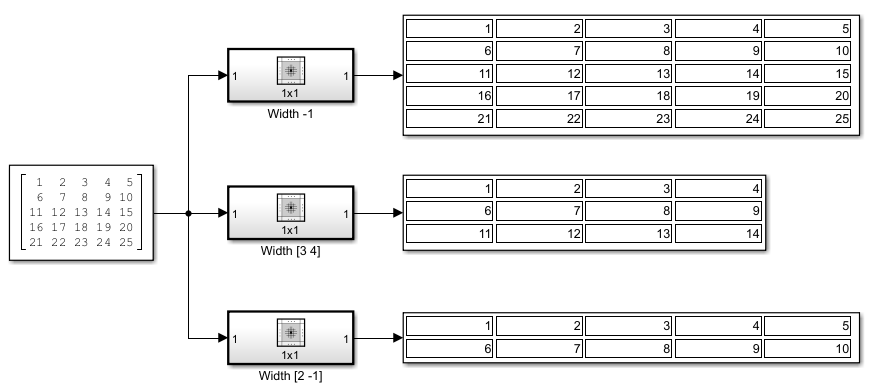Use the Processing offset and Processing width parameters together to define the top left and bottom right boundaries of a region of interest. For an example, see Specify Region of Interest for Neighborhood Processing.

#### Programmatic Use

 Block Parameter: `ProcessingWidth` Type: array Values: `-1` | `''` Default: `-1`

Configure which ROI elements you want the Neighborhood Processing Subsystem block to iterate over by selecting the value `Same`, `Full`, or `Valid`. This determines the dimensions of the output matrix.

Each example graphic shows a 5-by-5 region of interest with three highlighted subsections.

• The blue top left subsection represents the neighborhood that the Neighborhood Processing Subsystem block processes in the first iteration.

• The orange bottom right subsection represents the neighborhood of the last iteration.

• The green center subsection represents the dimensions of the output matrix, which spans the centers of the first and last iterations.

For settings that use padded values, the example graphics denote padded values as `?`.

Each example graphic assumes a Neighborhood size parameter value of `[3 3]`, which defines a 3-by-3 neighborhood. Because each example graphic assumes the default value of `[0 0]` for the Processing offset parameter and the default value of `-1` for the Processing width parameter, the region of interest is the full input matrix. For information about how the Neighborhood Processing Subsystem block pads values when you configure a region of interest, see Use Neighborhood Processing Subsystem Block Padding Options with Region of Interest.

• `Same` –– The output matrix has the same dimensions as the region of interest. For elements near the edges, the neighborhood extends beyond the input matrix. Use the Padding option parameter to configure how to pad elements outside the input matrix.• `Full` –– The output matrix is larger than the region of interest. The matrix includes an element for every neighborhood that includes at least one element from the region of interest, even if the neighborhood center is outside the region of interest. Use the Padding option parameter to configure how to pad elements outside the input matrix.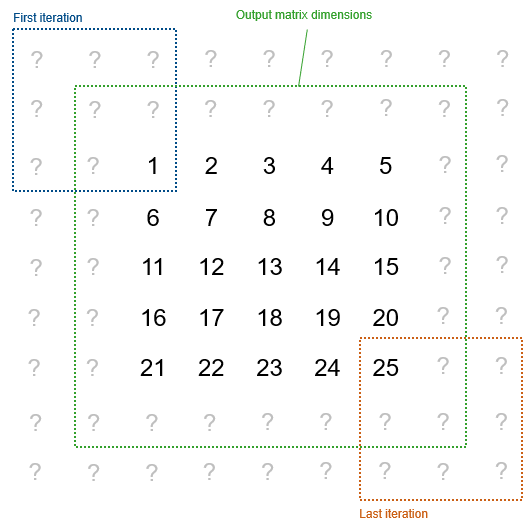• `Valid` –– The output matrix is smaller than the region of interest. The output matrix includes only the elements whose neighborhoods fall entirely within the region of interest.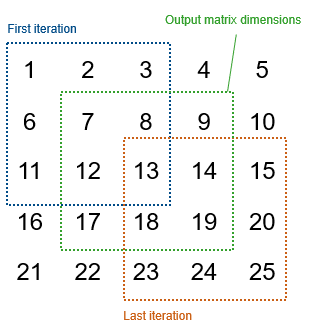#### Programmatic Use

 Block Parameter: `OutputSize` Type: character vector Values: `'Same'` | `'Full'` | `'Valid'` Default: `'Same'`

Configure how to pad values outside the input matrix. When you select the value `Full` or `Same` for the Output size parameter, some neighborhoods extend beyond the input matrix and use padded matrix element values.

To fully illustrate the logic of each padding option, each example graphic shows the 5-by-5 input matrix padded with 5-by-5 matrices. In practice, not every padded value is necessarily part of a neighborhood, depending on the Neighborhood size and Output size parameters. For example, if you set Neighborhood size to `[3 3]` and Output size to `Same`, the subsystem uses only the innermost layer of padded values.

• `Constant` –– Use a configurable constant value for elements outside the input matrix. For example, this matrix uses a constant value of `0`.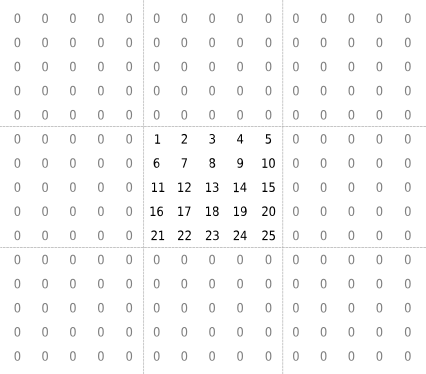.

• `Replicate` –– Extend the values of the edge parameters.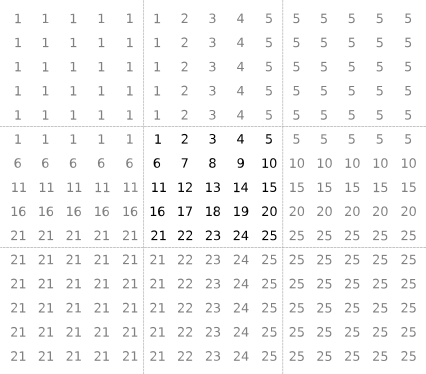.

• `Symmetric` –– Mirror the input matrix.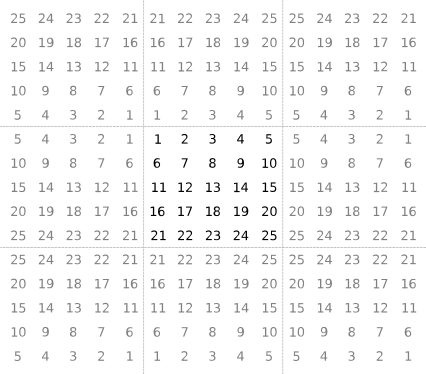.

• `Circular` –– Repeat the input matrix in a tiled pattern.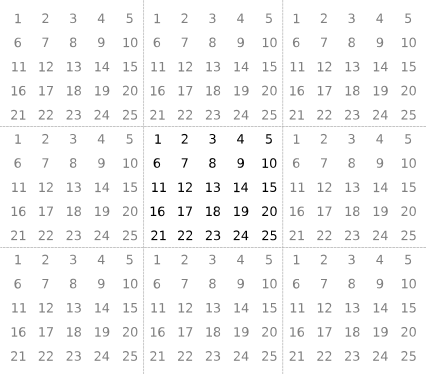.

For information about how the Padding option parameter interacts with the Processing offset and Processing width parameters, see Use Neighborhood Processing Subsystem Block Padding Options with Region of Interest.

#### Programmatic Use

 Block Parameter: `PaddingOption` Type: character vector Values: `'Constant'` | `'Replicate'` | `'Symmetric'` | `'Circular'` Default: `'Constant'`

#### Dependencies

To enable this parameter, set Output size to `Same` or `Full`.

Configure the value to use for the `Constant` padding option.

#### Dependencies

To enable this parameter, set Padding option to `Constant`.

#### Programmatic Use

 Block Parameter: `PaddingConstant` Type: scalar Values: `0` | `''` Default: `0`

## Version History

Introduced in R2022b